# Multi-dimensional distribution

multivariate distribution

A probability distribution on the-algebra of Borel sets of an-dimensional Euclidean space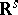. One usually speaks of a multivariate distribution as the distribution of a multi-dimensional random variable, or random vector,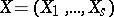, meaning by this the joint distribution of the real random variablesgiven on the same space of elementary events(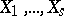may be regarded as coordinate variables in the space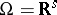). A multivariate distribution is uniquely determined by its distribution function — the function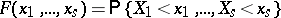of the real variables.

As in the one-dimensional case, the most widespread multivariate distributions are the discrete and the absolutely-continuous distributions. In the discrete case a multivariate distribution is concentrated on a finite or countable set of pointsofsuch that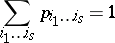(see, for example, Multinomial distribution). In the absolutely-continuous case almost-everywhere (with respect to Lebesgue measure) on,where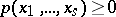is the density of the multivariate distribution: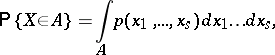for anyfrom the-algebra of Borel subsets of, and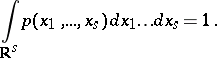The distribution of any random variable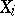(and also, for any, the distribution of the variables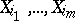) relative to a multivariate distribution is called a marginal distribution. The marginal distributions are completely determined by the given multivariate distribution. Whenare independent, then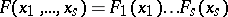and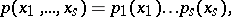where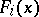and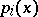are, respectively, the marginal distribution functions and densities of the.

The mathematical expectation of any function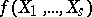ofis defined by the integral of this function with respect to the multivariate distribution; in particular, in the absolutely-continuous case it is defined by the integral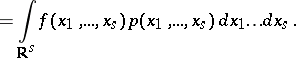The characteristic function of a multivariate distribution is the function of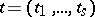given by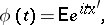where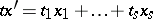. The fundamental characteristics of a multivariate distribution are the moments (cf. Moment): the mixed moments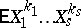and the central mixed moments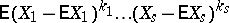, whereis the order of the corresponding moment. The roles of the expectation and the variance for a multivariate distribution are played by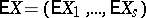and the set of second-order central mixed moments, which form the covariance matrix. If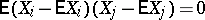for all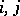,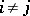, thenare called pairwise uncorrelated or orthogonal (the covariance matrix is diagonal). If the rankof the covariance matrix is less than, then the multivariate distribution is called a degenerate distribution; in this case the distribution is concentrated on some linear manifold inof dimension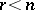.

For methods of investigating dependencies betweensee Correlation; Regression.